##### Microeconomics For Dummies - UKThe Stackelberg model of oligopoly within managerial economics illustrates one firm’s leadership in an oligopoly. In the Stackelberg model, the leader decides how much output to produce with other firms basing their decision on what the leader chooses.

Another common form of leadership is for the leading firm to set price. Rival firms then use the same price for their products. However, as is always the case in oligopoly, the leading firm must take into account the behavior of its rivals.

The leading firm that initially sets price is called the dominant firm. The firms that use the price set by the dominant firm are typically smaller in size and called following firms. Markets for steel and agricultural implements have been observed to operate in this manner.

The theory of price leadership represents a combination of monopoly behavior on the dominant firm’s part and perfectly competitive behavior on the part of following firms.

The illustration below shows price leadership. The market has a downward-sloping demand curve labeled D. A crucial point: The dominant firm must take into account that its following firms satisfy part of that market demand. Because the following firms act as price takers, their marginal revenue curve is the price set by the dominant firm.

To maximize profits, the following firms produce where price/marginal revenue equals marginal cost. Therefore, each following firm’s supply curve corresponds to its marginal cost curve, and the aggregate supply curve for all following firms is the horizontal summation of the marginal cost curves. This horizontal summation of marginal cost is represented by the curve ”MCf.

In the illustration, the dominant firm’s demand curve, dd, is derived from the market demand and the aggregate supply curve for the following firms. The dominant firm simply subtracts the quantity provided by the following firms from the quantity demanded in the market to determine its quantity demanded.

In other words, the dominant firm’s demand curve equals the market demand curve minus the sum of the following firm’s marginal cost curves, ”MCf.

Given the linear relationships, the dominant firm’s marginal revenue curve, MRd, is twice as steep as its demand curve. The dominant firm maximizes profit by producing the quantity of output that corresponds to marginal revenue, MRd, equals marginal cost, MCd. This output level is qd.

The dominant firm determines price by going from the quantity qd up to its demand curve, dd. Thus, the dominant firm sets the price at Pd.

As already noted, following firms are price takers. The dominant firm’s price Pd is the price that all following firms charge for every unit of the product they sell. For the following firms, the dominant firm’s price becomes their marginal revenue, Pd = MRf. The following firms then maximize profit by setting marginal revenue, MRf, equal to marginal cost, MCf. In aggregate, the following firms produce qf of output.

For the market, consumers pay a price of Pd and consume the quantity Q. The quantity consumed Q equals qd plus qf.

The market demand for an oligopoly characterized by price leadership is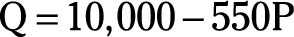where Q is the market quantity demanded and P is the good’s price in dollars.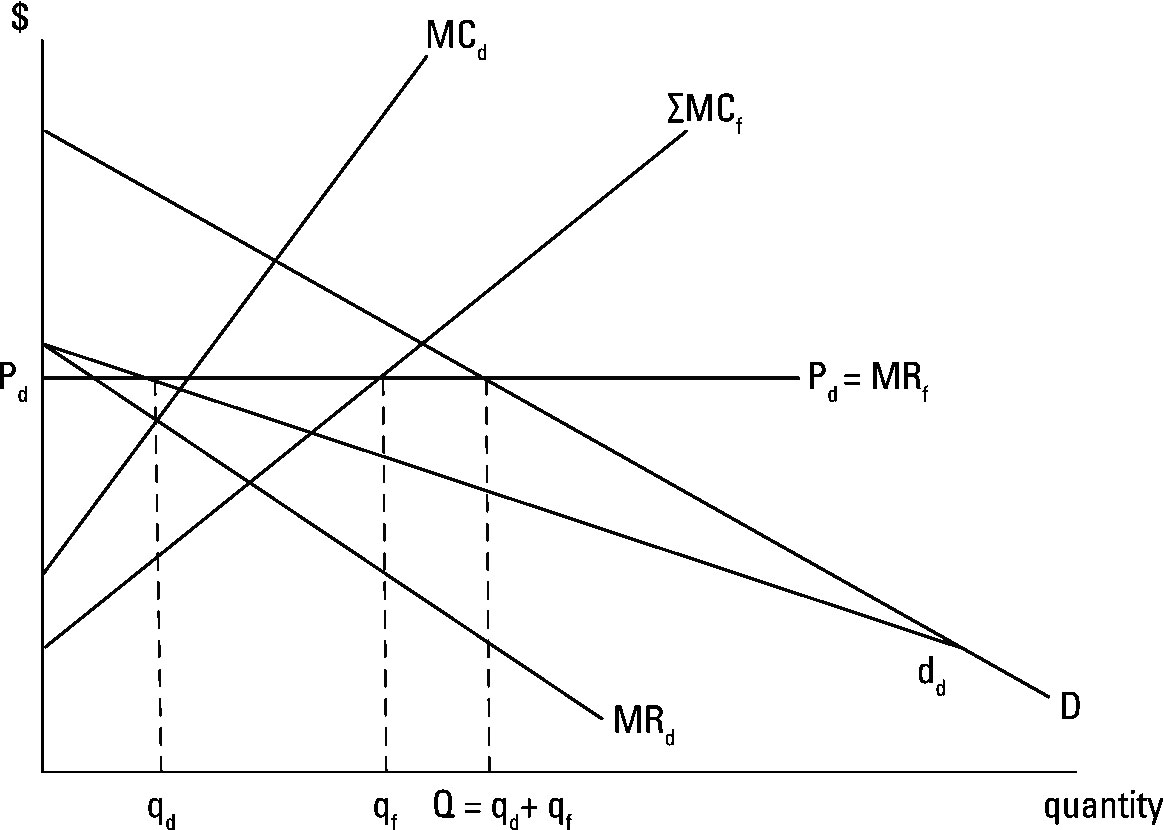The aggregate marginal cost for the following firms is represented by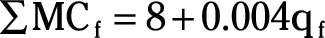where ”MCf is the horizontal summation of marginal cost for the following firms and qf is the aggregate quantity produced by the following firms.

The dominant firm’s marginal cost curve is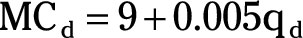where MCd is the dominant firm’s marginal cost in dollars and qd is the quantity produced by the dominant firm.

In order to determine the good’s market price and the quantity of the good produced by the dominant firm and the following firms, you take the following steps:

1. Derive the dominant firm’s demand curve.

Note that the market quantity demanded Q equals: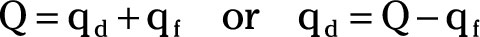2. Rearrange the following firms’ aggregate marginal cost curve to get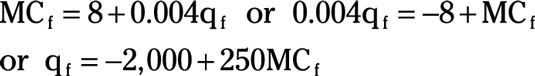3. Substitute P for MCf in the equation from Step 2.

This substitution is allowed because following firms produce where price equals marginal cost in order to maximize profit.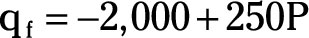4. In the Step 1 equation, substitute the market demand equation for Q and the equation for qf from Step 3.

This step generates the equation for the dominant firm’s demand curve.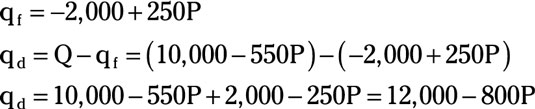5. Rearrange the equation in Step 4 to solve for P as a function of qd.

This form is converted to the total revenue equation in the next step.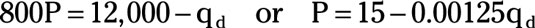6. Determine the dominant firm’s total revenue equation.

Remember, total revenue equals price multiplied by quantity.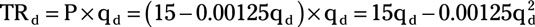7. Determine the dominant firm’s marginal revenue equation.

Take the derivative of total revenue with respect to qd.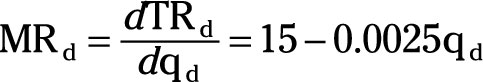8. Determine the dominant firm’s profit-maximizing quantity of output.

Set the dominant firm’s marginal revenue equal to the dominant firm’s marginal cost and solve for qd.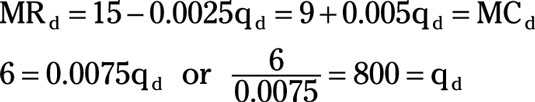9. Substitute qd equals 800 into the dominant firm’s demand curve in order to determine the price established by the dominant firm.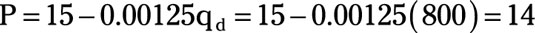The dominant firm produces 800 units of output and charges a price of \$14.

10. Determine the following firms aggregate quantity of output.

Following firms are price takers, so the dominant firm’s price is the following firms’ marginal revenue.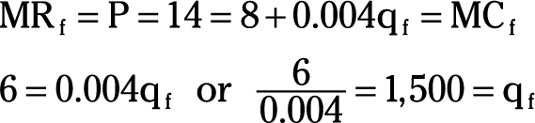11. Determine the market quantity demanded.

Substitute 14 for price P in the market demand equation.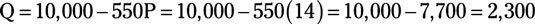So the dominant firm produces 800 units of output at a price of \$14. The following firms produce an aggregate of 1,500 units. The market quantity demanded given a price of \$14 is 2,300 units — the same as qd plus qf.

In the price leadership model, only the dominant firm has monopoly power — only the dominant firm can set price.